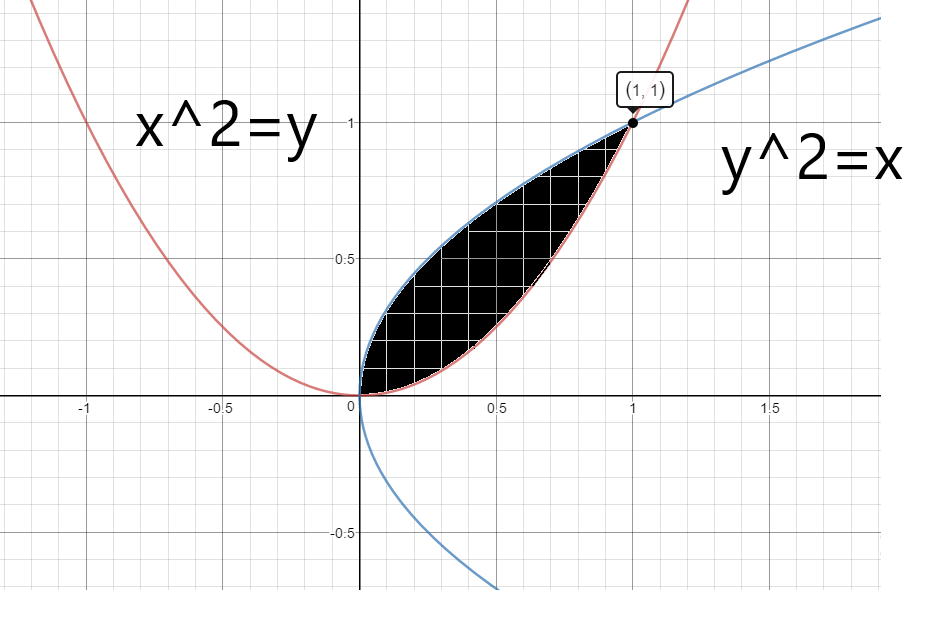QUESTION

# Find the area of the region bounded by the two parabolas $y = {x^2}$and ${y^2} = x$.

Hint – First we draw the sketch of the curves and find the region which is common for both curves, shaded the area bounded by the curves, and then find the area of that shaded region.

Complete step-by-step solution -
Given in the question two parabolas, $y = {x^2}$and ${y^2} = x$.
The sketch of the given parabolas is given below-To find the point of intersection of the two curves, take the equations $y = {x^2}$ and ${y^2} = x$.
Taking, ${y^2} = x$
Put $y = {x^2}$ in the above equation, we get-
${x^4} = x \\ \Rightarrow {x^4} - x = 0 \\ \Rightarrow x({x^3} - 1) = 0 \\ \Rightarrow x = 0,1 \\$
For x = 0, y = 0.
For x =1, y = 1.
Hence, the points of intersection of the two parabolas are (0,0) and (1,1).
From the graph we can see the shaded region bounded by the two curves.
Thus, the required area = area of the shaded region.
$A = |\int\limits_0^1 {({x^2} - \sqrt x } )dx| = |\dfrac{{{x^3}}}{3} - \dfrac{2}{3}{x^{\dfrac{3}{2}}}|_0^1 \\ A = |\dfrac{1}{3} - \dfrac{2}{3}| = \dfrac{1}{3}sq.unit \\$

Note – Whenever such types of questions appear, always find the point of intersection of the parabolas, and then shade the region bounded by the curve. To find the area of the shaded region, integrate the equation of the curve in the shaded region.